Probability Topics

# Independent and Mutually Exclusive Events

Independent and mutually exclusive do not mean the same thing.

### Independent Events

Two events are independent if the following are true:

• P(A|B) = P(A)
• P(B|A) = P(B)
• P(A AND B) = P(A)P(B)

Two events A and B are independent if the knowledge that one occurred does not affect the chance the other occurs. For example, the outcomes of two roles of a fair die are independent events. The outcome of the first roll does not change the probability for the outcome of the second roll. To show two events are independent, you must show only one of the above conditions. If two events are NOT independent, then we say that they are dependent.

Sampling may be done with replacement or without replacement.

• With replacement: If each member of a population is replaced after it is picked, then that member has the possibility of being chosen more than once. When sampling is done with replacement, then events are considered to be independent, meaning the result of the first pick will not change the probabilities for the second pick.
• Without replacement: When sampling is done without replacement, each member of a population may be chosen only once. In this case, the probabilities for the second pick are affected by the result of the first pick. The events are considered to be dependent or not independent.

If it is not known whether A and B are independent or dependent, assume they are dependent until you can show otherwise.

You have a fair, well-shuffled deck of 52 cards. It consists of four suits. The suits are clubs, diamonds, hearts and spades. There are 13 cards in each suit consisting of 1, 2, 3, 4, 5, 6, 7, 8, 9, 10, J (jack), Q (queen), K (king) of that suit.

a. Sampling with replacement:
Suppose you pick three cards with replacement. The first card you pick out of the 52 cards is the Q of spades. You put this card back, reshuffle the cards and pick a second card from the 52-card deck. It is the ten of clubs. You put this card back, reshuffle the cards and pick a third card from the 52-card deck. This time, the card is the Q of spades again. Your picks are {Q of spades, ten of clubs, Q of spades}. You have picked the Q of spades twice. You pick each card from the 52-card deck.

b. Sampling without replacement:
Suppose you pick three cards without replacement. The first card you pick out of the 52 cards is the K of hearts. You put this card aside and pick the second card from the 51 cards remaining in the deck. It is the three of diamonds. You put this card aside and pick the third card from the remaining 50 cards in the deck. The third card is the J of spades. Your picks are {K of hearts, three of diamonds, J of spades}. Because you have picked the cards without replacement, you cannot pick the same card twice.

Try It

You have a fair, well-shuffled deck of 52 cards. It consists of four suits. The suits are clubs, diamonds, hearts and spades. There are 13 cards in each suit consisting of 1, 2, 3, 4, 5, 6, 7, 8, 9, 10, J (jack), Q (queen), K (king) of that suit. Three cards are picked at random.

1. Suppose you know that the picked cards are Q of spades, K of hearts and Q of spades. Can you decide if the sampling was with or without replacement?
2. Suppose you know that the picked cards are Q of spades, K of hearts, and J of spades. Can you decide if the sampling was with or without replacement?

You have a fair, well-shuffled deck of 52 cards. It consists of four suits. The suits are clubs, diamonds, hearts, and spades. There are 13 cards in each suit consisting of 1, 2, 3, 4, 5, 6, 7, 8, 9, 10, J (jack), Q (queen), and K (king) of that suit. S = spades, H = Hearts, D = Diamonds, C = Clubs.

1. Suppose you pick four cards, but do not put any cards back into the deck. Your cards are QS, 1D, 1C, QD.
2. Suppose you pick four cards and put each card back before you pick the next card. Your cards are KH, 7D, 6D, KH.

Which of a. or b. did you sample with replacement and which did you sample without replacement?

a. Without replacement; b. With replacement

Try It

You have a fair, well-shuffled deck of 52 cards. It consists of four suits. The suits are clubs, diamonds, hearts, and spades. There are 13 cards in each suit consisting of 1, 2, 3, 4, 5, 6, 7, 8, 9, 10, J (jack), Q (queen), and K (king) of that suit. S = spades, H = Hearts, D = Diamonds, C = Clubs. Suppose that you sample four cards without replacement. Which of the following outcomes are possible? Answer the same question for sampling with replacement.

1. QS, 1D, 1C, QD
2. KH, 7D, 6D, KH
3. QS, 7D, 6D, KS

### Mutually Exclusive Events

A and B are mutually exclusive events if they cannot occur at the same time. This means that A and B do not share any outcomes and P(A AND B) = 0.

For example, suppose the sample space S = {1, 2, 3, 4, 5, 6, 7, 8, 9, 10}. Let A = {1, 2, 3, 4, 5}, B = {4, 5, 6, 7, 8}, and C = {7, 9}. A AND B = {4, 5}. P(A AND B) =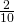and is not equal to zero. Therefore, A and B are not mutually exclusive. A and C do not have any numbers in common so P(A AND C) = 0. Therefore, A and C are mutually exclusive.

If it is not known whether A and B are mutually exclusive, assume they are not until you can show otherwise. The following examples illustrate these definitions and terms.

Flip two fair coins. (This is an experiment.)

The sample space is {HH, HT, TH, TT} where T = tails and H = heads. The outcomes are HH, HT, TH, and TT. The outcomes HT and TH are different. The HT means that the first coin showed heads and the second coin showed tails. The TH means that the first coin showed tails and the second coin showed heads.

• Let A = the event of getting at most one tail. (At most one tail means zero or one tail.) Then A can be written as {HH, HT, TH}. The outcome HH shows zero tails. HT and TH each show one tail.
• Let B = the event of getting all tails. B can be written as {TT}. B is the complement of A, so B = A′. Also, P(A) + P(B) = P(A) + P(A′) = 1.
• The probabilities for A and for B are P(A) =and P(B) =.
• Let C = the event of getting all heads. C = {HH}. Since B = {TT}, P(B AND C) = 0. B and C are mutually exclusive. (B and C have no members in common because you cannot have all tails and all heads at the same time.)
• Let D = event of getting more than one tail. D = {TT}. P(D) =• Let E = event of getting a head on the first roll. (This implies you can get either a head or tail on the second roll.) E = {HT, HH}. P(E) =• Find the probability of getting at least one (one or two) tail in two flips. Let F = event of getting at least one tail in two flips. F = {HT, TH, TT}. P(F) =Try It

Draw two cards from a standard 52-card deck with replacement. Find the probability of getting at least one black card.

Flip two fair coins. Find the probabilities of the events.

1. Let F = the event of getting at most one tail (zero or one tail).
2. Let G = the event of getting two faces that are the same.
3. Let H = the event of getting a head on the first flip followed by a head or tail on the second flip.
4. Are F and G mutually exclusive?
5. Let J = the event of getting all tails. Are J and H mutually exclusive?

Look at the sample space in (Figure).

1. Zero (0) or one (1) tails occur when the outcomes HH, TH, HT show up. P(F) =2. Two faces are the same if HH or TT show up. P(G) =3. A head on the first flip followed by a head or tail on the second flip occurs when HH or HT show up. P(H) =4. F and G share HH so P(F AND G) is not equal to zero (0). F and G are not mutually exclusive.
5. Getting all tails occurs when tails shows up on both coins (TT). H’s outcomes are HH and HT.

J and H have nothing in common so P(J AND H) = 0. J and H are mutually exclusive.

Try It

A box has two balls, one white and one red. We select one ball, put it back in the box, and select a second ball (sampling with replacement). Find the probability of the following events:

1. Let F = the event of getting the white ball twice.
2. Let G = the event of getting two balls of different colors.
3. Let H = the event of getting white on the first pick.
4. Are F and G mutually exclusive?
5. Are G and H mutually exclusive?

Roll one fair, six-sided die. The sample space is {1, 2, 3, 4, 5, 6}. Let event A = a face is odd. Then A = {1, 3, 5}. Let event B = a face is even. Then B = {2, 4, 6}.

• Find the complement of A, A′. The complement of A, A′, is B because A and B together make up the sample space. P(A) + P(B) = P(A) + P(A′) = 1. Also, P(A) =and P(B) =.
• Let event C = odd faces larger than two. Then C = {3, 5}. Let event D = all even faces smaller than five. Then D = {2, 4}. P(C AND D) = 0 because you cannot have an odd and even face at the same time. Therefore, C and D are mutually exclusive events.
• Let event E = all faces less than five. E = {1, 2, 3, 4}.

Are C and E mutually exclusive events? (Answer yes or no.) Why or why not?

No. C = {3, 5} and E = {1, 2, 3, 4}. P(C AND E) =. To be mutually exclusive, P(C AND E) must be zero.

• Find P(C|A). This is a conditional probability. Recall that the event C is {3, 5} and event A is {1, 3, 5}. To find P(C|A), find the probability of C using the sample space A. You have reduced the sample space from the original sample space {1, 2, 3, 4, 5, 6} to {1, 3, 5}. So, P(C|A) =.
Try It

Let event A = learning Spanish. Let event B = learning German. Then A AND B = learning Spanish and German. Suppose P(A) = 0.4 and P(B) = 0.2. P(A AND B) = 0.08. Are events A and B independent? Hint: You must show ONE of the following:

• P(A|B) = P(A)
• P(B|A) = P(B)
• P(A AND B) = P(A)P(B)

P(A|B) =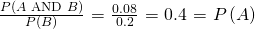The events are independent because P(A|B) = P(A).

Let event G = taking a math class. Let event H = taking a science class. Then, G AND H = taking a math class and a science class. Suppose P(G) = 0.6, P(H) = 0.5, and P(G AND H) = 0.3. Are G and H independent?

If G and H are independent, then you must show ONE of the following:

• P(G|H) = P(G)
• P(H|G) = P(H)
• P(G AND H) = P(G)P(H)
NOTE

The choice you make depends on the information you have. You could choose any of the methods here because you have the necessary information.

a. Show that P(G|H) = P(G).

P(G|H) =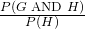=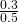= 0.6 = P(G)

b. Show P(G AND H) = P(G)P(H).

P(G)P(H) = (0.6)(0.5) = 0.3 = P(G AND H)

Since G and H are independent, knowing that a person is taking a science class does not change the chance that he or she is taking a math class. If the two events had not been independent (that is, they are dependent) then knowing that a person is taking a science class would change the chance he or she is taking math. For practice, show that P(H|G) = P(H) to show that G and H are independent events.

Try It

In a bag, there are six red marbles and four green marbles. The red marbles are marked with the numbers 1, 2, 3, 4, 5, and 6. The green marbles are marked with the numbers 1, 2, 3, and 4.

• R = a red marble
• G = a green marble
• O = an odd-numbered marble
• The sample space is S = {R1, R2, R3, R4, R5, R6, G1, G2, G3, G4}.

S has ten outcomes. What is P(G AND O)?

Let event C = taking an English class. Let event D = taking a speech class.

Suppose P(C) = 0.75, P(D) = 0.3, P(C|D) = 0.75 and P(C AND D) = 0.225.

1. Are C and D independent?
2. Are C and D mutually exclusive?
3. What is P(D|C)?
1. Yes, because P(C|D) = P(C).
2. No, because P(C AND D) is not equal to zero.
3. P(D|C) =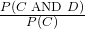=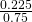= 0.3
Try It

A student goes to the library. Let events B = the student checks out a book and D = the student checks out a DVD. Suppose that P(B) = 0.40, P(D) = 0.30 and P(B AND D) = 0.20.

1. Find P(B|D).
2. Find P(D|B).
3. Are B and D independent?
4. Are B and D mutually exclusive?

In a box there are three red cards and five blue cards. The red cards are marked with the numbers 1, 2, and 3, and the blue cards are marked with the numbers 1, 2, 3, 4, and 5. The cards are well-shuffled. You reach into the box (you cannot see into it) and draw one card.

Let R = red card is drawn, B = blue card is drawn, E = even-numbered card is drawn.

The sample space S = R1, R2, R3, B1, B2, B3, B4, B5. S has eight outcomes.

• P(R) =. P(B) =. P(R AND B) = 0. (You cannot draw one card that is both red and blue.)
• P(E) =. (There are three even-numbered cards, R2, B2, and B4.)
• P(E|B) =. (There are five blue cards: B1, B2, B3, B4, and B5. Out of the blue cards, there are two even cards; B2 and B4.)
• P(B|E) =. (There are three even-numbered cards: R2, B2, and B4. Out of the even-numbered cards, to are blue; B2 and B4.)
• The events R and B are mutually exclusive because P(R AND B) = 0.
• Let G = card with a number greater than 3. G = {B4, B5}. P(G) =. Let H = blue card numbered between one and four, inclusive. H = {B1, B2, B3, B4}. P(G|H) =. (The only card in H that has a number greater than three is B4.) Since=, P(G) = P(G|H), which means that G and H are independent.
Try It

• 70% of the fans are rooting for the home team.
• 25% of the fans are wearing blue.
• 20% of the fans are wearing blue and are rooting for the away team.
• Of the fans rooting for the away team, 67% are wearing blue.

Let A be the event that a fan is rooting for the away team.
Let B be the event that a fan is wearing blue.
Are the events of rooting for the away team and wearing blue independent? Are they mutually exclusive?

In a particular college class, 60% of the students are female. Fifty percent of all students in the class have long hair. Forty-five percent of the students are female and have long hair. Of the female students, 75% have long hair. Let F be the event that a student is female. Let L be the event that a student has long hair. One student is picked randomly. Are the events of being female and having long hair independent?

• The following probabilities are given in this example:
• P(F) = 0.60; P(L) = 0.50
• P(F AND L) = 0.45
• P(L|F) = 0.75
NOTE

The choice you make depends on the information you have. You could use the first or last condition on the list for this example. You do not know P(F|L) yet, so you cannot use the second condition.

Solution 1Check whether P(F AND L) = P(F)P(L). We are given that P(F AND L) = 0.45, but P(F)P(L) = (0.60)(0.50) = 0.30. The events of being female and having long hair are not independent because P(F AND L) does not equal P(F)P(L).

Solution 2Check whether P(L|F) equals P(L). We are given that P(L|F) = 0.75, but P(L) = 0.50; they are not equal. The events of being female and having long hair are not independent.

Interpretation of ResultsThe events of being female and having long hair are not independent; knowing that a student is female changes the probability that a student has long hair.

Try It

Mark is deciding which route to take to work. His choices are I = the Interstate and F = Fifth Street.

• P(I) = 0.44 and P(F) = 0.56
• P(I AND F) = 0 because Mark will take only one route to work.

What is the probability of P(I OR F)?

1. Toss one fair coin (the coin has two sides, H and T). The outcomes are ________. Count the outcomes. There are ____ outcomes.
2. Toss one fair, six-sided die (the die has 1, 2, 3, 4, 5 or 6 dots on a side). The outcomes are ________________. Count the outcomes. There are ___ outcomes.
3. Multiply the two numbers of outcomes. The answer is _______.
4. If you flip one fair coin and follow it with the toss of one fair, six-sided die, the answer in part c. is the number of outcomes (size of the sample space). What are the outcomes? (Hint: Two of the outcomes are H1 and T6.)
5. Event A = heads (H) on the coin followed by an even number (2, 4, 6) on the die.
A = {_________________}. Find P(A).
6. Event B = heads on the coin followed by a three on the die. B = {________}. Find P(B).
7. Are A and B mutually exclusive? (Hint: What is P(A AND B)? If P(A AND B) = 0, then A and B are mutually exclusive.)
8. Are A and B independent? (Hint: Is P(A AND B) = P(A)P(B)? If P(A AND B) = P(A)P(B), then A and B are independent. If not, then they are dependent).
1. H and T; 2
2. 1, 2, 3, 4, 5, 6; 6
3. 2(6) = 12
4. T1, T2, T3, T4, T5, T6, H1, H2, H3, H4, H5, H6
5. A = {H2, H4, H6}; P(A) =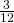6. B = {H3}; P(B) =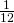7. Yes, because P(A AND B) = 0
8. P(A AND B) = 0.P(A)P(B) =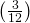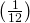. P(A AND B) does not equal P(A)P(B), so A and B are dependent.
Try It

A box has two balls, one white and one red. We select one ball, put it back in the box, and select a second ball (sampling with replacement). Let T be the event of getting the white ball twice, F the event of picking the white ball first, S the event of picking the white ball in the second drawing.

1. Compute P(T).
2. Compute P(T|F).
3. Are T and F independent?.
4. Are F and S mutually exclusive?
5. Are F and S independent?

### References

Lopez, Shane, Preety Sidhu. “U.S. Teachers Love Their Lives, but Struggle in the Workplace.” Gallup Wellbeing, 2013. http://www.gallup.com/poll/161516/teachers-love-lives-struggle-workplace.aspx (accessed May 2, 2013).

Data from Gallup. Available online at www.gallup.com/ (accessed May 2, 2013).

### Chapter Review

Two events A and B are independent if the knowledge that one occurred does not affect the chance the other occurs. If two events are not independent, then we say that they are dependent.

In sampling with replacement, each member of a population is replaced after it is picked, so that member has the possibility of being chosen more than once, and the events are considered to be independent. In sampling without replacement, each member of a population may be chosen only once, and the events are considered not to be independent. When events do not share outcomes, they are mutually exclusive of each other.

### Formula Review

If A and B are independent, P(A AND B) = P(A)P(B), P(A|B) = P(A) and P(B|A) = P(B).

If A and B are mutually exclusive, P(A OR B) = P(A) + P(B) and P(A AND B) = 0.

E and F are mutually exclusive events. P(E) = 0.4; P(F) = 0.5. Find P(EF).

J and K are independent events. P(J|K) = 0.3. Find P(J).

P(J) = 0.3

U and V are mutually exclusive events. P(U) = 0.26; P(V) = 0.37. Find:

1. P(U AND V) =
2. P(U|V) =
3. P(U OR V) =

Q and R are independent events. P(Q) = 0.4 and P(Q AND R) = 0.1. Find P(R).

P(Q AND R) = P(Q)P(R)

0.1 = (0.4)P(R)

P(R) = 0.25

### Homework

Use the following information to answer the next 12 exercises. The graph shown is based on more than 170,000 interviews done by Gallup that took place from January through December 2012. The sample consists of employed Americans 18 years of age or older. The Emotional Health Index Scores are the sample space. We randomly sample one Emotional Health Index Score.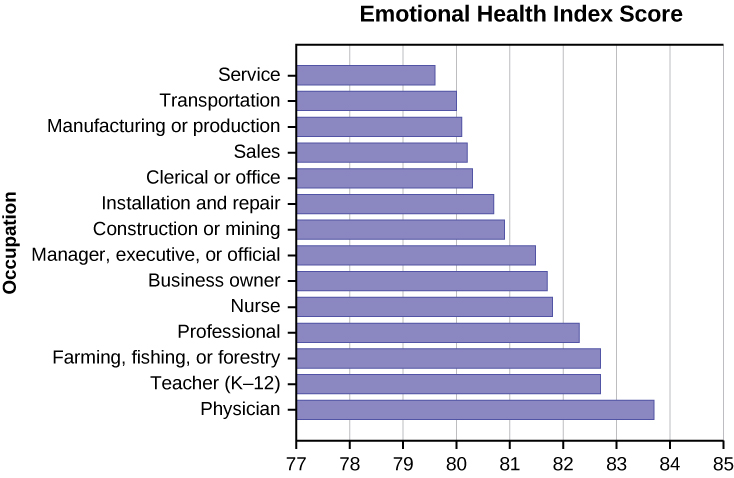Find the probability that an Emotional Health Index Score is 82.7.

Find the probability that an Emotional Health Index Score is 81.0.

0

Find the probability that an Emotional Health Index Score is more than 81?

Find the probability that an Emotional Health Index Score is between 80.5 and 82?

0.3571

If we know an Emotional Health Index Score is 81.5 or more, what is the probability that it is 82.7?

What is the probability that an Emotional Health Index Score is 80.7 or 82.7?

0.2142

What is the probability that an Emotional Health Index Score is less than 80.2 given that it is already less than 81.

What occupation has the highest emotional index score?

Physician (83.7)

What occupation has the lowest emotional index score?

What is the range of the data?

83.7 − 79.6 = 4.1

Compute the average EHIS.

If all occupations are equally likely for a certain individual, what is the probability that he or she will have an occupation with lower than average EHIS?

P(Occupation < 81.3) = 0.5

### Bringing It Together

A previous year, the weights of the members of the San Francisco 49ers and the Dallas Cowboys were published in the San Jose Mercury News. The factual data are compiled into (Figure).

Shirt# ≤ 210 211–250 251–290 290≤
1–33 21 5 0 0
34–66 6 18 7 4
66–99 6 12 22 5

For the following, suppose that you randomly select one player from the 49ers or Cowboys.

If having a shirt number from one to 33 and weighing at most 210 pounds were independent events, then what should be true about P(Shirt# 1–33|≤ 210 pounds)?

The probability that a male develops some form of cancer in his lifetime is 0.4567. The probability that a male has at least one false positive test result (meaning the test comes back for cancer when the man does not have it) is 0.51. Some of the following questions do not have enough information for you to answer them. Write “not enough information” for those answers. Let C = a man develops cancer in his lifetime and P = man has at least one false positive.

1. P(C) = ______
2. P(P|C) = ______
3. P(P|C’) = ______
4. If a test comes up positive, based upon numerical values, can you assume that man has cancer? Justify numerically and explain why or why not.
1. P(C) = 0.4567
2. not enough information
3. not enough information
4. No, because over half (0.51) of men have at least one false positive text

Given events G and H: P(G) = 0.43; P(H) = 0.26; P(H AND G) = 0.14

1. Find P(H OR G).
2. Find the probability of the complement of event (H AND G).
3. Find the probability of the complement of event (H OR G).

Given events J and K: P(J) = 0.18; P(K) = 0.37; P(J OR K) = 0.45

1. Find P(J AND K).
2. Find the probability of the complement of event (J AND K).
3. Find the probability of the complement of event (J OR K).
1. P(J OR K) = P(J) + P(K) − P(J AND K); 0.45 = 0.18 + 0.37 – P(J AND K); solve to find P(J AND K) = 0.10
2. P(NOT (J AND K)) = 1 – P(J AND K) = 1 – 0.10 = 0.90
3. P(NOT (J OR K)) = 1 – P(J OR K) = 1 – 0.45 = 0.55

### Glossary

Dependent Events
If two events are NOT independent, then we say that they are dependent.
Sampling with Replacement
If each member of a population is replaced after it is picked, then that member has the possibility of being chosen more than once.
Sampling without Replacement
When sampling is done without replacement, each member of a population may be chosen only once.
The Conditional Probability of One Event Given Another Event
P(A|B) is the probability that event A will occur given that the event B has already occurred.
The OR of Two Events
An outcome is in the event A OR B if the outcome is in A, is in B, or is in both A and B.